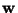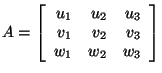Next: Example 1 Up: Applications of Determinant Previous: Hint

## Volume of a a parallelepiped

Suppose three vectorsandin three dimensional spaceare given so that they do not lie in the same plane. These three vectors form three edges of a parallelepiped. The volume of this parallelepiped ( is the product of area of the base and altitude ) is equal to the scalar triple product.

It can be shown that the volume of the parallelepiped is the absolute value of the determinant of the following matrix:.

Subsections